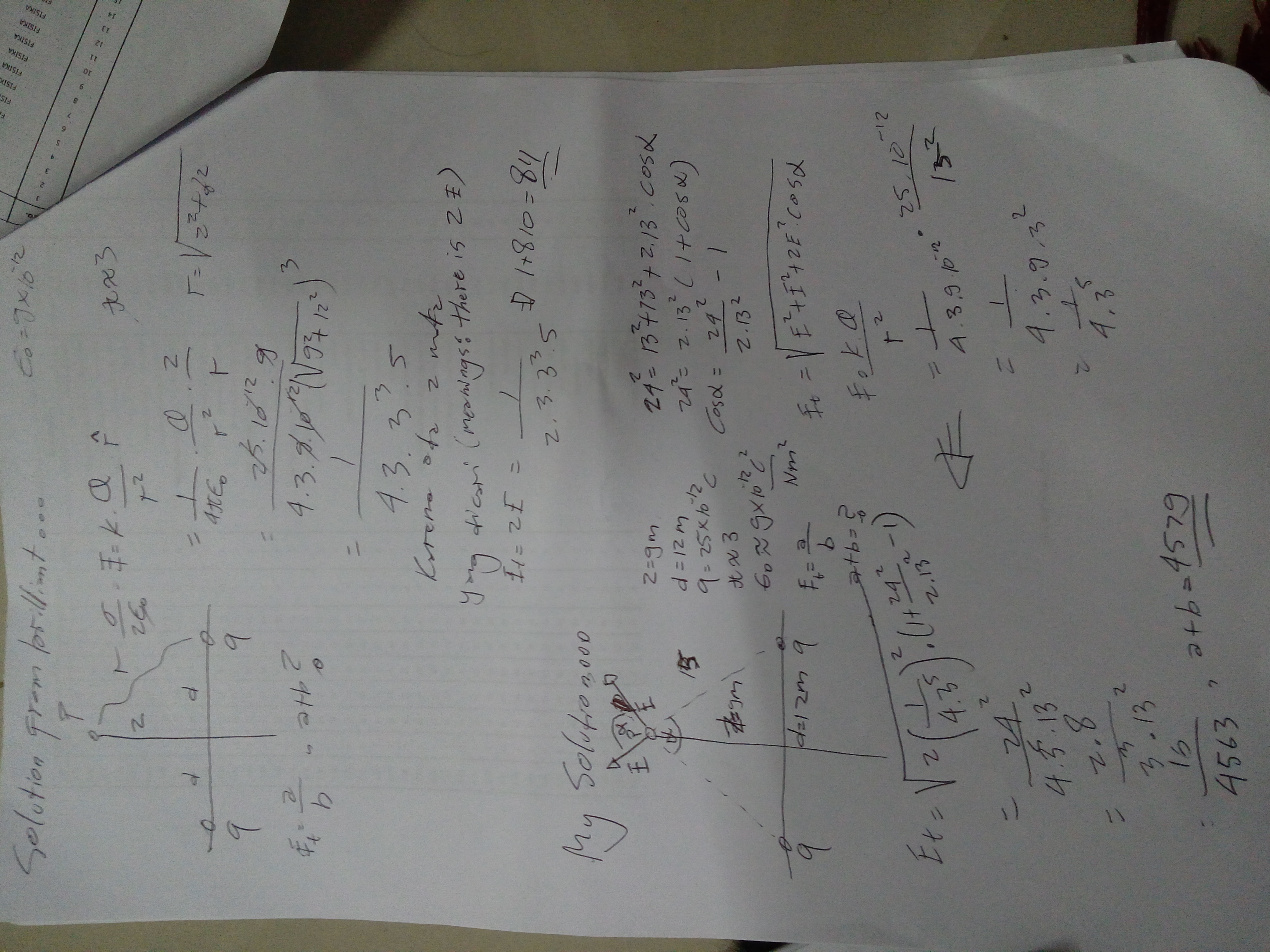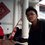Why this Brilliant solution's true and i wrong?

i never know that the formula of electic field is ${E}=\frac{1}{4\pi\epsilon_0}\frac{Q}{r^2}\hat{r}$.

From many books i have read event in this wiki they never mentioned $\hat{r}$.

I need an explaination about this. Anyway i don't understand this Brilliant solution. Can anybody explain ! why this Brilliant solution's true and i wrong?

(sorry for the pic, if you can't see it clearly feel free to download and rotate it)Note by Hafizh Ahsan Permana
4 years, 6 months ago

This discussion board is a place to discuss our Daily Challenges and the math and science related to those challenges. Explanations are more than just a solution — they should explain the steps and thinking strategies that you used to obtain the solution. Comments should further the discussion of math and science.

When posting on Brilliant:

• Use the emojis to react to an explanation, whether you're congratulating a job well done , or just really confused .
• Ask specific questions about the challenge or the steps in somebody's explanation. Well-posed questions can add a lot to the discussion, but posting "I don't understand!" doesn't help anyone.
• Try to contribute something new to the discussion, whether it is an extension, generalization or other idea related to the challenge.
• Stay on topic — we're all here to learn more about math and science, not to hear about your favorite get-rich-quick scheme or current world events.

MarkdownAppears as
*italics* or _italics_ italics
**bold** or __bold__ bold

- bulleted
- list

• bulleted
• list

1. numbered
2. list

1. numbered
2. list
Note: you must add a full line of space before and after lists for them to show up correctly
paragraph 1

paragraph 2

paragraph 1

paragraph 2

> This is a quote
This is a quote
# I indented these lines
# 4 spaces, and now they show
# up as a code block.

print "hello world"
# I indented these lines
# 4 spaces, and now they show
# up as a code block.

print "hello world"
MathAppears as
Remember to wrap math in $$...$$ or $...$ to ensure proper formatting.
2 \times 3 $2 \times 3$
2^{34} $2^{34}$
a_{i-1} $a_{i-1}$
\frac{2}{3} $\frac{2}{3}$
\sqrt{2} $\sqrt{2}$
\sum_{i=1}^3 $\sum_{i=1}^3$
\sin \theta $\sin \theta$
\boxed{123} $\boxed{123}$

Sort by:

Hey @Hafizh Ahsan Permana! Can you link to the problem that you're trying to solve? I'd be happy to help.

Regarding the reason why $E = \frac{1}{4\pi\varepsilon_0}\frac{1}{r^2}$, the simplest is that it is what's measured experimentally. Reality is the thing that decides what theories in science are right or wrong, so it's pretty much the end of the road as far as Coulomb's law goes.

On a slightly deeper level, you might consider the electric field to radiate out from charged particles. In fact, if you go further on in physics, you learn that all forces are carried by a particle. In other words, the electric force is brought about by the charges exchange of photons. This gives some basis to think of the charge as sending out uniformly electric field in all directions. Then, just as the intensity of light from the Sun or a light bulb diminishes as you walk away from it, so goes the electric field of a charged particle. This line of thinking brings you to Gauss' law. I think Gauss' law is probably the closest it gets to "understanding" why Coulomb's law has the form that it does.

Staff - 4 years, 5 months ago

Hello @Josh Silverman! No, i can't link to the problem because it's practice problem. The data in the problem is already written in the picture above and i think it's enough to solve the problem. Actually my solution had many failures in counting, Do you think my solution will work and result the same divisor? @Siddhartha Srivastava said it will work but i can't prove it. I don't know, maybe its my failure again in my counting or it's really not same but has close result.

- 4 years, 5 months ago

A few things.

• The Cosine rule is $C^2 = A^2 + B^2 - AB\cos(\theta)$. There is a $-$ on $AB\cos(\theta)$. Not a $+$ sign.

• Where did you get the $13$ in the cosine rule from? The hypotenuse is $15$, not $13$.

• You should raise your doubts in the question's solution discussion. It'll make it easier for everyone to find out what the problem is since they've just solved it and know the question. I took me some time just to figure out the question.

Reply to this post once you read this so that I can delete my comment. Also delete your note. If you have any other problems, reply with the link to the question and ask your question in the solution discussion of the problem.

- 4 years, 6 months ago

No. I can't ask it in the solution discussion because it's on practice and no one can discuss this there. Brilliant suggested me to post it on note. Thanks for the correction but can my solution really worked? I confused, i have no doubt with the solution, i just don't understand the solution is, i never seen the solution in any books and i need some expert explanation. Once again thanks for the comment.

- 4 years, 6 months ago

Sorry, my bad. I thought it was a community question. Anyways, your solution should work. Your method is a little long, but correct.

The Brilliant solution is nothing new or different. It just exploits the symmetry of the problem. By symmetry, you can see that the horizontal components of the electric field of each of the particles cancel out. The only thing that is left is the vertical components. What is the vertical component of the Electric field of one component? $\dfrac{z}{R} \times E$. That is where the $\dfrac{z}{R}$ came from. Since there are two particles, you multiply by 2.

- 4 years, 6 months ago

Happy to know that my solution can really work! And your explaination helped my understanding. It will be completed if there's more problem and solutions like this. Do you know this kind of questions? and the questions can work by this solution. Not only electric field, anything. Because Practice Question only give me one example. Well if i repeat the practice then will come the same question but different numbers.

- 4 years, 6 months ago

Since Electric field is a vector quantity, it needs some unit vector to represent it.

- 4 years, 6 months ago

I don't understand why it is converted as z/r. Do you have any problem and solution like this? (Different picture)

- 4 years, 6 months ago